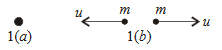# Consider the following two statementsA         Linear momentum of a system of particles is zero.B         Kinetic energy of a system of particles is zero.Then Option 1) A does not imply B and B does not imply A Option 2) A  implies B but B does not imply A Option 3) A does not imply B but B  implies A Option 4) A implies imply B and  B  implies A

As we learnt in

Kinetic energy -

- wherein

kinetic Energy is frame dependentLinear momentum is a vector quantity linear momentum of a system of particle is zero does not means that momentum of each particle is zero.

i.e. each particle has momentum but their vector sum is zero.

Kinetic energy is scaler quantity hence its summation is n ot zero even if linear momentum is zero.

If kinetic energy of system of particle is zero means kinetic enery of each particle is zero.

In this case total linear momentum is zero.

This means B implies A but A doesnot implies B.

Option 1)

A does not imply B and B does not imply A

Option 2)

A  implies B but B does not imply A

Option 3)

A does not imply B but B  implies A

Option 4)

A implies imply B and  B  implies A

N

### Preparation Products

##### JEE Main Rank Booster 2021

This course will help student to be better prepared and study in the right direction for JEE Main..

₹ 13999/- ₹ 9999/-
##### Knockout JEE Main April 2021 (Subscription)

An exhaustive E-learning program for the complete preparation of JEE Main..

₹ 6999/- ₹ 5/-
##### Knockout JEE Main April 2021

An exhaustive E-learning program for the complete preparation of JEE Main..

₹ 22999/- ₹ 14999/-
##### Knockout JEE Main April 2022

An exhaustive E-learning program for the complete preparation of JEE Main..

₹ 34999/- ₹ 24999/-